4.4.2 Characteristic Constraints

The constraints characteristic of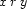or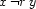for each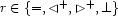can be easily expressed in terms of the node representations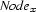and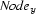.

proc {Equal N1 N2} N1=N2 end
proc {NotEqual N1 N2}
{FS.disjoint N1.eq N2.eq}
end
proc {Above N1 N2}
{FS.subset N2.eqdown N1.down}
{FS.subset N1.equp N2.up}
{FS.subset N1.side N2.side}
end
proc {NotAbove N1 N2}
{FS.disjoint N1.eq N2.up}
{FS.disjoint N2.eq N1.down}
end
proc {Disjoint N1 N2}
{FS.subset N1.eqdown N2.side}
{FS.subset N2.eqdown N1.side}
end
proc {NotDisjoint N1 N2}
{FS.disjoint N1.eq N2.side}
{FS.disjoint N2.eq N1.side}
end

Denys Duchier
Version 1.2.0 (20010221)